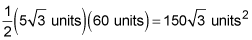##### Geometry: 1,001 Practice Problems For Dummies (+ Free Online Practice)If you are asked to find the area of a regular polygon, you can do so by using a formula that includes the perimeter of the polygon and a measurement called the apothem.

The apothem is the line segment from the center of the polygon to the midpoint of one of the sides, and is perpendicular to that side. The perimeter is the total distance around the polygon.

The formula for the area of a regular polygon is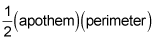## Practice questions

1. Find the area of a regular pentagon whose perimeter is 40 units and whose apothem is 5 units.

2. Find the exact area of a regular hexagon that has a perimeter of 60 units.

1. 100 units2

The formula for the area of a regular polygon isThe apothem is 5 and the perimeter is 40, so the area is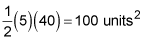2.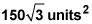The formula for the area of a regular polygon isA regular hexagon is a polygon with six equal sides. You're given that the perimeter of the hexagon is 60 units, which means each side is 10. The apothem is joined to the midpoint of one of the sides and is also perpendicular to the side, forming a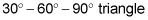The side opposite the 30-degree angle is x, the side opposite the 60-degree angle is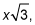and the side opposite the 90-degree angle is 2x. The apothem is opposite the 60-degree angle, so the apothem equals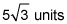When you plug everything into the formula, you get